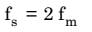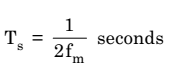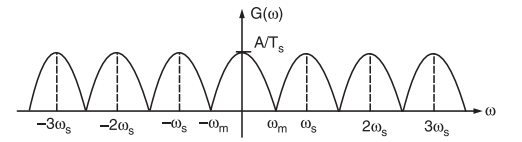# What is Nyquist Rate and Nyquist Interval

## Nyquist Rate

When the sampling rate becomes exactly equal to 2fm samples per second, then it is called Nyquist rate. Nyquist rate is also called the minimum sampling rate. It is given by,Similarly, maximum sampling interval is called Nyquist interval. It is given by,When the continuous-time band-limited signal is sampled at Nyquist rate (fs = 2fm), the sampled-spectrum G(ω) contains non-overlapping G(ω) repeating periodically.

But the successive cycles of G(ω) touch each other as shown in fig.1. Therefore, the original spectrum X(ω) can be recovered from the sampled spectrum by using a low pass filter (LPF) with a cut-off frequency ωm.Fig.1: Sampled spectrum at Nyquist rate